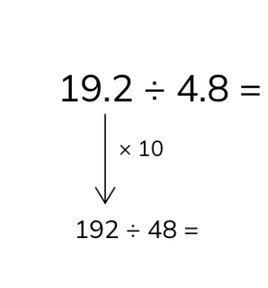Dividing two decimal numbers

# Dividing two decimal numbers

Students learn to divide two decimal numbers.8,000 schools use Gynzy92,000 teachers use Gynzy1,600,000 students use Gynzy

## General

The students learn to divide a decimal number with 1 or 2 decimal places by another decimal number.

## Standards

CCSS.Math.Content.5.NBT.B.7

## Learning objective

Students will be able to divide two decimal numbers with 1 or 2 decimal places.

## Introduction

Show the story problems on the interactive whiteboard and have the students solve them.

## Instruction

First explain how you can divide a decimal number with one decimal place. You can do this by first ensuring that the problem does not have any decimal numbers. By multiplying both numbers in the problem by 10, you solve away the decimals. After that you solve the problem without decimals. You can use a helping row to help solve the problem. Explain that the problems will have the same answer with and without the decimals. You can divide numbers with two decimal places in the same way. Multiply by 100 to solve away the decimals. Have the students practice this with several problems with decimal numbers with one or two decimal places. Finally you explain how you solve a story problem and have the students solve a few problems in story form.

Check whether the students can divide decimal numbers by another decimal number by asking the following questions:
- What steps do you take to solve a problem with decimal numbers?
- How do you solve the following problem: 14.1 ÷ 4.7?

## Quiz

The students test their understanding of dividing two decimal numbers through ten exercises. In some of these exercises they must divide numbers with one decimal place, and in others they must divide numbers with two decimal places. Some of the exercises are story problems.

## Closing

You discuss once again with the students how it is important to be able to divide a decimal number with one or two decimal places by another decimal, because that is how you know how much everyone gets if you want to divide something up evenly. Next you divide the class into six teams. Each team takes a turn throwing the die and moves that number of steps with their placeholder. Next the team solves the problem on the interactive whiteboard. If their answer is wrong, they take a step back with their placeholder. If their answer is correct, they stay where they are. Then it is the next team's turn. Keep going like this until one team reaches the end of the board. You can drag each of the cards away to reveal the following problem.

## Teaching tips

Students that have difficulty with dividing a decimal number by another decimal can first practice dividing larger numbers without decimal points. Next you explain that the problem 12.64 ÷ 3.16 is the same as 1,264 ÷ 316. By solving away the decimal point, you can then solve the problem. Explain that the decimal point moves one place to the right if you multiply by 10 and two places if you multiply by 100.

## Instruction materials

A die to use in the closing activity

### The online teaching platform for interactive whiteboards and displays in schools

• Save time building lessons

• Manage the classroom more efficiently

• Increase student engagement